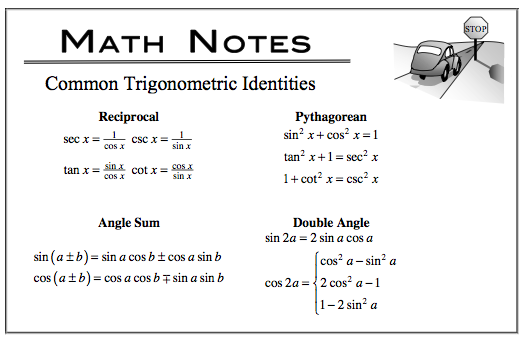### Home > CALC > Chapter 6 > Lesson 6.3.4 > Problem6-117

6-117.

Multiple Choice: Which of the following expressions is equal to $\frac { d } { d x } ( \operatorname { cos } ^ { 2 } x )$?

1. $2\operatorname{cos} x$

1. $−2\operatorname{cos} x\operatorname{sin} x$

1. $−\operatorname{sin} 2x$

1. I only

1. II only

1. III only

1. II and III

1. none

There may be more than one correct answer.